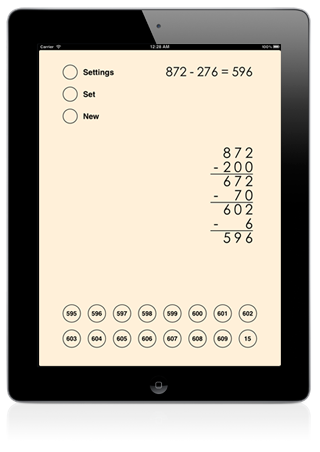# Left-to-Right Subtraction

### for iPad, iPhone, and Windows 10 PCs

This app can be used to teach and study the left-to-right subtraction method. The app is easy to use and it has an intuitive interactive interface with customizable colors and other settings. The user can solve custom and random subtraction problems and each step is made clearer with an animation.

##The left-to-right subtraction method

The left-to-right subtraction method is a two-step process. In the example 936 - 581, the number to be subtracted is first written in an expanded form: 500+80+1. Each of the parts is then subtracted from the starting number. In our example 936-500=436, 436-80=356 and finally 356-1=355.

In the Everyday Mathematics curriculum the left-to-right subtraction method is one of the algorithms for subtraction.

## The app is easy to use

Each step of the multi-step process is animated and the numbers are aligned automatically so that the user can concentrate on solving the operations for each step.

After you solve each sub-operation the correct answer will fly to the right place. If you press the wrong button the answer will appear above the keyboard but it will not move.

## Difficulty can be set

• The numbers can have 2 or 3 digits.
• The current operation can be hidden.
• The operands of the current operation can be highlighted.
• Colors of the interface can be changed.
• The speed of the animations can be set.

84 - 17

283 - 195

## Left-to-Right Subtraction in the Apple VPP Store for Education

The Volume Purchase Program allows participating educational institutions to purchase iDevBooks math apps in volume and distribute them to students. All iDevBooks math apps offer special 50% discount for purchases of 20 apps or more for participating educational institutions.

Left-to-Right Subtraction in the VPP store